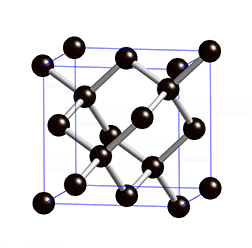## Bandstructure of germanium (Ge)

The electron bandstructure for germanium (diamond structure) was calculated using the program Quantum Espresso (version 5.3.0). Ge is a semiconductor with a direct bandgap of 0.67 eV. The calculated bandgap was adjusted to get the right gap. The course of the calculated bands correlates to comparative bandstructures for Ge (cf. MaterialsDesign) .Annotation:
The outcoming data of Quantum Espresso were prepared by a Matlab-Script. The script allows to choose a order of symmetry points and plots the corresponding bandstructure. Also the density of states can be plotted.

Matlab-Script:
Input files:

Output files:
Ge_data.zipDiamond structure of Ge Source: Wikipedia

Density of states